# Electronics and Communication Engineering - Exam Questions Papers

1.

If we manage to __________ our natural resources, we would leave a better planet for our children.

 A. uphold B. restrain C. Cherish D. conserve

Explanation:

The clue in this sentence is 'If we manage to __________ our natural resources' and 'better planet'.

This implies that the blank should be filled by a word which means 'preserve' or 'keep for long time'.

Therefore the word 'conserve' is the right answer.

2.

(2)3 + (3)4 = (?)5

 A. 4 B. 11 C. none of these D. not possible

Explanation:

(2)3 + (3)4 = 2 + 3 = 5 and (10)5 = 51 + 0 = 5

(2)3 + (3)4 = (10)5 .

3.

If g(t) = e- p t2 then G(0) is __________ (where g(t)⇔ G(f)

 A.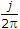B. unity C.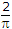D. impulse at f = 0

Explanation:

The signal g(t) given above is a Gaussian pulse, and it satisfies the relation.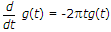Therefore, its fourier transform is same as the signal itself in frequency domain.

i.e. G(f) = e-pf2

Hence G(f)|f = 0 = e-p(0) = 1.

4.

The open loop transfer function of a unity feedback system is: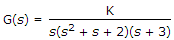The range of K for which the system is stable is

 A.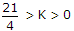B. 13 > K > 0 C.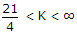D. -6 < K < ∞

Explanation:

Apply R-H.

5.

A discrete time linear shift-invariant system has an impulse response h[n] with h = 1, h = - 1, h - 2, and zero otherwise. The system is given an input sequence x[n] with x - x - 1, and zero otherwise. The number of nonzero samples in the output sequence y[n], and the value of y are, respectively

 A. 5, 2 B. 6, 2 C. 6, 1 D. 5, 3